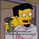253 vizualizações
The Semi Deviation Point calculates the positive value as the standard deviation of only samples that has high value than the simple moving average . The negative value is the same standard deviation, but only uses the low value and multiply for -1.

After this calculate the value is divide by the value of simple moving average and multiplied by hundred to get a percent value from 0% to 100%.

The delta line is a simple moving average from the differences of the two values.

If positive values are greater than negative values than the volatility is happen on higher prices and not lower.
If positive values are lower than negative values than the volatility is happen on lower prices and not higher.

If you know that in which market you would opt in? Is it less risk the prior?

## ComentáriosThanks, modified your script to use volume information
src = volume
delta = abs(dp - semi)
Works really well at finding exhaustion points, combine this with an entry point and you've got a winner

Resposta